• matlab深度学习语音降噪案例，此示例说明如何使用深度学习网络对语音信号去噪。该示例比较应用于同一任务的两种类型的网络：全连接网络和卷积网络。
• 针对语音通信中不可避免要受到各种噪声的影响,致使通信质量下降的问题,采用DSPTMS320C5509和语音采集芯片TLV320AIC23搭建了语音降噪系统,实现了基于谱相减技术的实时系统来消除环境噪声。通过DSP开发板对信号进行...
• 今天小编就为大家分享一篇Python谱减法语音降噪实例，具有很好的参考价值，希望对大家有所帮助。一起跟随小编过来看看吧
• 采用kalman滤波算法，对含噪声的语音信号进行降噪，能够取得较好的效果。
• 一篇关于讲解语音降噪的方法的硕士论文，对于做语音信号处理很有参考意义。
• 语音信号的免费降噪 该库提供了语音信号降噪的能力。 它已被实现以一种方式抑制噪声语音信号系统（即没有噪声信号参考源的系统）。 它对于在嘈杂环境中工作且具有单个麦克风的电话终端可能很有用。 注意：此噪声抑制...
• 双信号转换LSTM网络 Tensorflow 2.x实施的堆叠式双信号转换LSTM网络（DTLN）用于实时噪声抑制。 该存储库提供了用于在python中训练，推断和服务DTLN模型的代码。 它还提供了SavedModel，TF-lite和ONNX格式的预训练...
• 摘 要： 针对语音通信中不可避免要受到各种噪声的影响，致使通信质量下降的问题，采用DSP TMS320C5509和语音采集芯片TLV320AIC23搭建了语音降噪系统，实现了基于谱相减技术的实时系统来消除环境噪声。通过DSP开发板...
• 摘 要： 针对语音通信中不可避免要受到各种噪声的影响，致使通信质量下降的问题，采用DSP TMS320C5509和语音采集芯片TLV320AIC23搭建了语音降噪系统，实现了基于谱相减技术的实时系统来消除环境噪声。通过DSP开发板...
• 语音降噪-维纳滤波维纳滤波原理时频域维纳滤波器频域维纳滤波器迭代维纳滤波器 维纳滤波原理 输入信号通过一个线性时不变系统之后产生一个输出信号,使得输出信号尽量逼近期望信号，使其估计误差最小化，能够最小化这...


语音降噪-维纳滤波
维纳滤波原理时频域维纳滤波器频域维纳滤波器语音降噪

维纳滤波原理
输入信号通过一个线性时不变系统之后产生一个输出信号,使得输出信号尽量逼近期望信号，使其估计误差最小化，能够最小化这个估计误差的最优滤波器称为维纳滤波器。
时频域维纳滤波器
假定输入信号

y

(

n

)

y(n)

和期望信号

d

(

n

)

d(n)

，定义估计的误差信号为

e

(

n

)

e(n)

e

(

n

)

=

d

(

n

)

−

d

^

(

n

)

=

d

(

n

)

−

h

T

y

e(n) = d(n) - \widehat{d}(n) \\=d(n) - \textbf{h}^T\textbf{y}

其中

h

T

=

[

h

0

,

h

1

,

.

.

.

,

h

M

−

1

]

\textbf{h}^T=[h_0,h_1,...,h_{M-1}]

是滤波器系数向量，

y

T

=

[

h

n

,

h

n

−

1

,

.

.

.

,

h

n

−

M

+

1

]

\textbf{y}^T=[h_{n},h_{n-1},...,h_{n-M+1}]

是输入向量。最小化

e

(

n

)

e(n)

的均方值,

J

=

E

[

e

2

(

n

)

]

=

E

(

d

(

n

)

−

h

T

y

)

2

=

E

[

d

2

(

n

)

]

+

h

T

E

[

y

y

T

]

h

−

2

h

T

E

[

d

(

n

)

y

]

=

E

[

d

2

(

n

)

]

+

h

T

R

y

y

h

−

2

h

T

r

y

d

J = E{[e^2(n)]}=E(d(n) - \mathbf{h}^T\mathbf{y})^2 \\=E{[d^2(n)]} + \mathbf{h}^TE[\mathbf{y}\mathbf{y}^T]\mathbf{h} - 2\mathbf{h}^TE{[d(n)\mathbf{y}]} \\=E{[d^2(n)]} + \mathbf{h}^TR_{yy}\mathbf{h} - 2\mathbf{h}^Tr_{\mathbf{y}d}

其中

r

y

d

r_{\textbf{y}d}

是输入和期望信号的互协方差矩阵，

R

y

y

R_{yy}

是输入信号的自协方差矩阵。 利用矩阵求导原理，求

J

J

对向量

h

h

的导数,得:

∂

J

∂

h

=

−

2

r

y

d

+

2

h

T

R

\frac{\partial J}{\partial \mathbf{h}} = -2 r_{\mathbf{y}d} + 2\mathbf{h}^TR

另导数为0，求得

h

∗

=

R

−

1

r

y

d

h^{*} = R^{-1}r_{\mathbf{y}d}

频域维纳滤波器
利用时域卷积等价于频域相乘的性质，可以得到误差的频域表达式为

E

(

w

k

)

=

D

(

w

k

)

−

D

^

(

w

k

)

=

D

(

w

k

)

−

H

(

w

k

)

Y

(

w

k

)

E(w_k) = D(w_k) - \widehat{D}(w_k) \\= D(w_k) - H(w_k) Y(w_k)

按照时域维纳滤波器一样的推导方式，可以得到最优维纳滤波器的频域表达式为

H

(

w

k

)

=

P

d

y

(

w

k

)

P

y

y

(

w

k

)

H(w_k) = \frac{P_{dy}(w_k)}{P_{yy}(w_k)}

其中

P

d

y

P_{dy}

是

y

(

n

)

y(n)

和

d

(

n

)

d(n)

的互功率谱，

P

y

y

P_{yy}

是

y

(

n

)

y(n)

的功率谱。
语音降噪
在语音降噪中，一般假设输入信号

y

(

n

)

y(n)

由干净语音

x

(

n

)

x(n)

和噪声信号

n

(

n

)

n(n)

组成，

y

(

n

)

=

x

(

n

)

+

n

(

n

)

y(n) = x(n) + n(n)

且假设噪声和语音不相关。 利用维纳滤波方法降噪时需要计算

R

y

y

R_{yy}

或者

P

y

y

P_{yy}

,由于假设噪声和语音不相关，则

R

y

y

=

R

x

x

+

R

n

n

P

y

y

=

P

x

x

+

P

n

n

R

y

x

=

R

x

x

P

y

x

=

P

x

x

R_{yy} = R_{xx} + R_{nn} \\P_{yy} = P_{xx} + P_{nn}\\R_{yx} = R_{xx} \\ P_{yx} = P_{xx}

最优的时频域滤波器估计可以分别表示为：

H

(

w

k

)

=

P

x

x

(

w

k

)

P

x

x

(

w

k

)

+

P

n

n

(

w

k

)

H(w_{k}) = \frac{P_{xx}(w_k)}{P_{xx}(w_k) + P_{nn}(w_k)}

h

=

R

x

x

R

x

x

+

R

n

n

\mathbf{h} = \frac{R_{xx}}{R_{xx} + R_{nn}}


展开全文信号处理
• 用户在进行音频通话时可能处于各种场景，嘈杂的背景声音对通话产生干扰，高质量的音频降噪能够有效提升用户通话体验。 QQ 团队基于开源 TensorFlow 机器学习平台实现了音频降噪、音质提升和模型优化算法，将降噪...腾讯 PCG QQ 团队 刘秋男  黄飞  石世昌  华超  杨梁
腾讯 QQ，8 亿人都在使用的即时通信软件，大量用户使用它进行免费视频、音频通话。用户在进行音频通话时可能处于各种场景，嘈杂的背景声音对通话产生干扰，高质量的音频降噪能够有效提升用户通话体验。
QQ 团队基于开源 TensorFlow 机器学习平台实现了音频降噪、音质提升和模型优化算法，将降噪算法应用于 QQ 音视频通话场景中，为用户提供 AI 赋能的智能通讯。

前言
传统降噪方法大部分结合数学、物理原理进行推导，其过程中难免基于人认知的理想先验假设，这降低了传统降噪方法对多种类、非平稳噪声的适应性。传统降噪因其计算量低，能够实时进行语音降噪的优势广为使用，但对实际场景中频发的多种类、非平稳噪声表现不佳。
AI 降噪是最近兴起的基于数据驱动的降噪方法，能够有效的应对各种突发的非平稳噪声，前提是需要大量的训练数据与合适的模型设计。模型在移动端上的部署需要权衡模型大小、降噪效果、CPU 占用率和内存占用率等多个因素，给降噪技术带来了一定的挑战。
本文基于 TensorFlow 平台搭建了一个 AI 降噪与噪声场景分类的并行训练框架，如 Fig.1 所示，打通了数据合成、模型搭建、训练推理及部署流程。此框架集中了 TensorFlow 众多 API，训练好模型后使用 TensorFlow Lite 转为 .tflite 文件。借助 TensorFlow Lite 的量化功能减小模型尺寸，在模型的降噪效果基本不受影响的前提下，极大提升了性能优势并成功部署到产品中上线。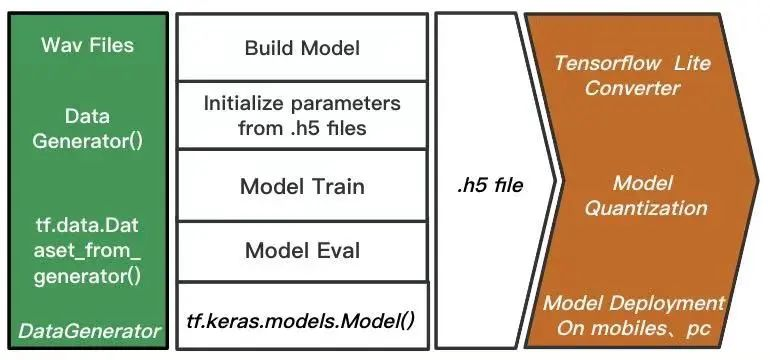Fig.1 基于 TensorFlow 的训练框图

训练框架搭建
数据处理
数据是以一边读取一边合成的方式动态合成带噪音频，数据读取和音频混合使用了 librosa、numpy 等开源框架，最后用 tf.data.Dataset.from_generator() 函数进行封装，模型在训练时直接调用此接口提取数据。
展示部分数据处理代码如下，自定义数据生成器 data_generator()，其中使用 librosa 开源框架读取音频为 numpy 数组格式，然后按照不同信噪比制作混合音频，每次 yield 一个样本，tf.data.Dataset_from_generator() 函数通过自身的迭代器与自定义的数据生成器遍历数据集。
def snr_mixer(clean_file, noise_file, snr, sample_rate=16000):
snr = np.random.randint(-5, 20)
speech, noise_ori, noisy = generate_mix_audio(clean_audio, noise_audio, snr)
return speech, noise_ori, noisy

def data_generator():
for file in self.clean_files:
idx = random.randint(0, len(self.noise_files) - 1)
noise_file = self.noise_names[idx]
speech, noise_ori, noisy = snr_mixer(file, noise_file, snr)
......
for index in range(num_blocks):
noisy_speech = noisy[.....]
clean_speech = speech[.....]
yield noisy_speech.astype('float32'), clean_speech.astype['float32']

dataset = tf.data.Dataset.from_generator(
data_generator,
(tf.float32, tf.float32),
output_shapes=(tf.TensorShape([]),tf.TensorShape([])))
dataset_val = tf.data.Dataset.from_generator(
...)

模型搭建
使用 tf.keras.layers 的各接口就可以快速的搭建模型，展示部分模型构建流程代码如下。模型的处理对象是时域语音信号，因此涉及到音频信号处理使用了 tf.signal 库。给定输入张量和输出张量，可以实例化一个 Model 类。
网络模型搭建完成后，需要对网络的学习过程进行配置，否则后续进行训练或者验证的时候会报错。可以使用 Model 类的成员函数 compile() 配置训练模型，可以自定义损失函数，或者使用库中自带损失函数，指定使用的优化器等。
模型配置完成后，调用 Model 类的成员函数 fit() 进行模型的训练。
from tf.keras.models import Model
from tf.keras.layers import Conv1D, Dense, LSTM, Input

noisy_audio = Input(batch_shape=(None, None), name='input_1')
windows = tf.signal.frame(noisy_audio, win_len, win_shift)
stft_res = tf.signal.rfft(windows)
....
istft_res = tf.signal.irfft(forward_res)
....
Output = ....
model = Model(inputs=noisy_audio, outputs=Output)

# Define Loss Function and optimizer
sgd = tf.keras.optimizers.SGD(lr=learning_rate, momentum=0.9)
model.compile(loss=self_define_loss(), optimizer=sgd)
model.fit(x=dataset,
epochs=20,
callbacks=[....],
validation_data=dataset_val)
模型部署及量化
TensorFlow Lite 是针对移动设备和嵌入式设备的轻量化模型推理解决方案，占用空间小，低延迟，具备在移动设备运行更快的内核解释器。
模型在训练时使用的数据是若干等时长的音频数据，为学习长时间音频的时序信息，模型中加入了 LSTM 结构。模型频域处理模块需要使用傅立叶正反变换计算，目前 TensorFlow Lite 还不支持 TensorFlow 中的傅立叶变换算子，但可以通过使用 TensorFlow  select 运算符来实现模型的转换。在考虑模型性能的情况下，我们重新实现了傅立叶正反变换算子，将模型以傅立叶正反变换为界分为两部分保存，针对我们的模型将傅立叶变换进行了简化加速。同时为保持流式处理与模型训练时的场景一致，流式处理的过程中会不断更新 LSTM 中的单元状态值，用于下一次输入音频的推理计算。
下面展示将模型切分保存为 .tflite 文件的流程，首先建立模型，加载训练好的模型参数；然后，建立 model_pre 和 model_aft，加载各自部分对应的模型参数；最后，定义 TensorFlow Lite 转换器(Converter)，将分别保存对应的 .tflite 文件。
model.load_weights(weights_file)
weights = model.get_weights()

model_pre = Model(inputs=[inp1, states_in_1], outputs=[out1, states_out_1])
model_aft = Model(inputs=[inp2, states_in_2],
outputs=[out2, states_out_2])

model_pre.set_weights(weights[:fir_parameter_nums])
model_aft.set_weights(weights[fir_parameter_nums:])

# 将前半部分模型转为 .tflite文件
converter = tf.lite.TFLiteConverter.from_keras_model(model_pre)
if Quantification:
converter.optimizations = [tf.lite.Optimize.DEFAULT]
tflite_model = converter.convert()
with tf.io.gfile.GFile(file_path + '_pre.tflite', 'wb') as f:
f.write(tflite_model)

# 将后半部分模型转为 .tflite文件
converter = tf.lite.TFLiteConverter.from_keras_model(model_aft)
if Quantification:
converter.optimizations = [tf.lite.Optimize.DEFAULT]
tflite_model = converter.convert()
with tf.io.gfile.GFile(file_path + '_2.tflite', 'wb') as f:
f.write(tflite_model)


TensorFlow Lite 提供训练后量化功能，通过设置优化标志针对模型尺寸进行优化，对浮点 TensorFlow 模型进行转换。对原 32 位模型进行 8 位量化后模型的大小降到原来的 1/4，对模型降噪效果几乎没有损害。Tab.1 为降噪模型部署在 Windows 开发机和 Mac 笔记本上时测量 CPU 占用率、内存占用等性能指标。
Tab.1  降噪模型的性能指标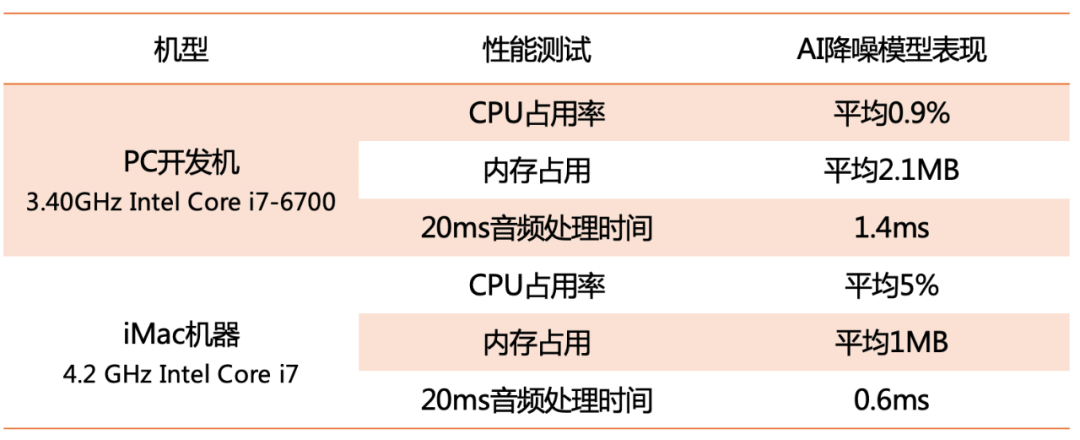音频降噪
新冠疫情的爆发让在线课堂、远程会议常态化，随之而来的则是一阵宠物嚎叫、孩子嘶吼、隔壁邻居的装修声等令人尴尬的场景。针对各种噪声场景进行合适的数据扩充能够提升模型的泛化能力，结合精细化设计的网络结构能够让 AI 降噪模型适应生活中常见的几百种噪声。
音频降噪模块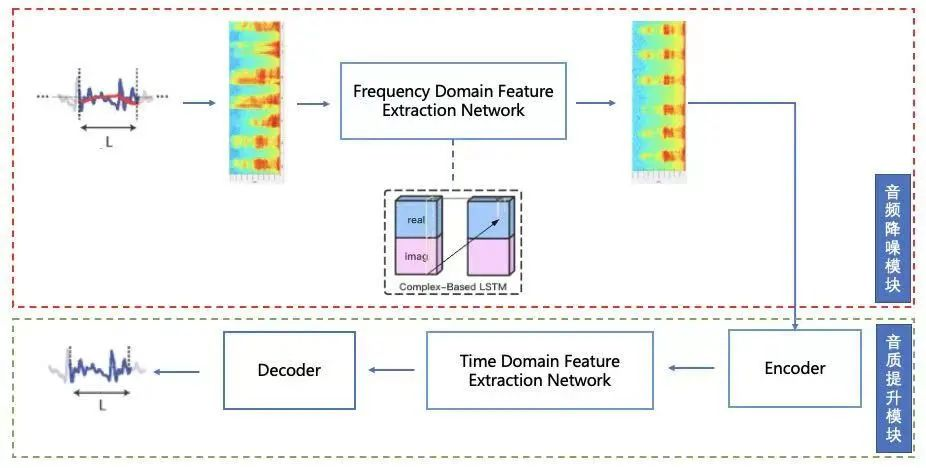Fig.2  AI 降噪模型结构

音质提升模块
音频降噪模块主要针对主讲人声音进行建模，认为其他的背景音如开门声、键盘声等都是噪声，可能会将有用的信息抹去的情况，听起来部分音频被消音。为了最大可能的避免这个情况，提升用户的通话体验，我们在音频降噪模块后面设计了音质提升模块。
该模块基于时域设计，沿用了使用较多的 Encoder-LSTM-Decoder 结构，Encoder、Decoder 部分以 Conv1D 卷积为主，时域特征提取模块以 LSTM 为主。

模型优化
用于音、视频通话中的降噪算法必须同时满足高质量降噪和实时处理两个高要求，设备计算力的限制往往会以牺牲降噪质量为代价，在这两大要求上同时追求极致成为了极大的挑战。为此，基于蒸馏技术进行模型优化。
在具体任务中模型参数量的增加能一定程度提高模型的表征学习能力，提升模型的指标效果，但受限于产品侧的各项性能要求，需要模型尽可能在有限参数量下达到指标评估最优。因此在保证模型性能（速度，内存占用）满足产品侧要求的条件下，为进一步提升模型效果，针对降噪模型设计和采用蒸馏策略，流程如 Fig.3 所示。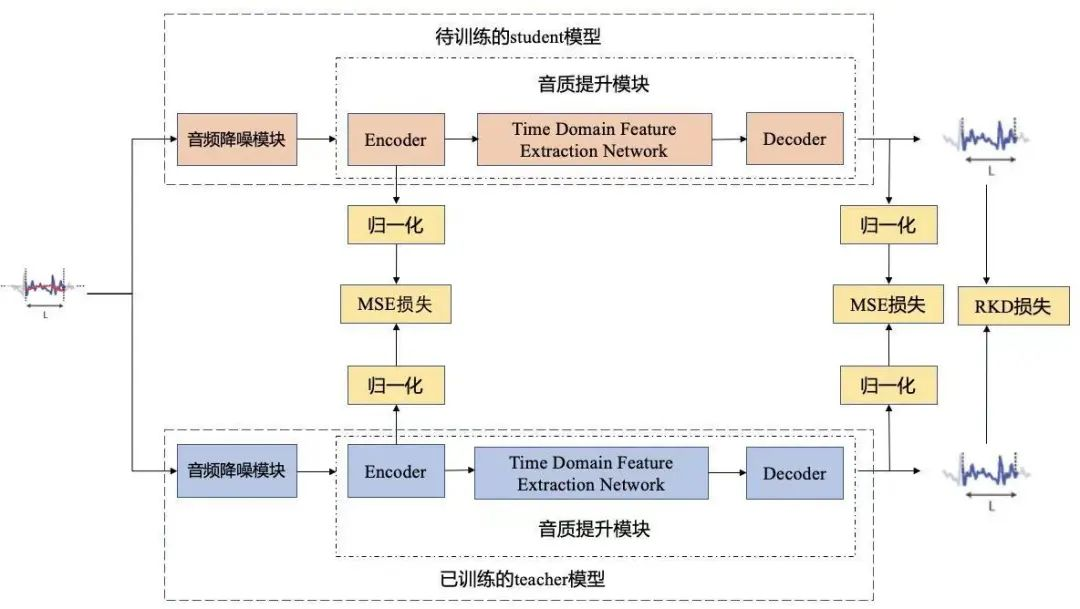Fig.3  降噪模型蒸馏流程

预训练比 student 模型大 10 倍左右参数量的 teacher 模型，全量数据训练下，teacher 模型要比 student 模型有更优的评估指标。针对音频去噪模型，在具有一定物理含义的编码层(Encoder)、解码层(Decoder)和降噪音频输出上，利用指标更优的 teacher 模型引导性能更优的 student 模型，从而在保证性能的同时进一步优化评估指标。

算法效果
时频域直观效果
如 Fig.4，分别从时域和频域的角度上观察音频降噪模块的输出音频和再经过音质提升模块的输出音频，音质提升模块的加入使噪声去除的更加干净。
音质提升模块是提升降噪效果的有效手段，精心设计的音质提升模块不会导致模型参数量的大幅上升，在可接受的性能影响范围内完全可以部署在用户手机当中，进行实时降噪。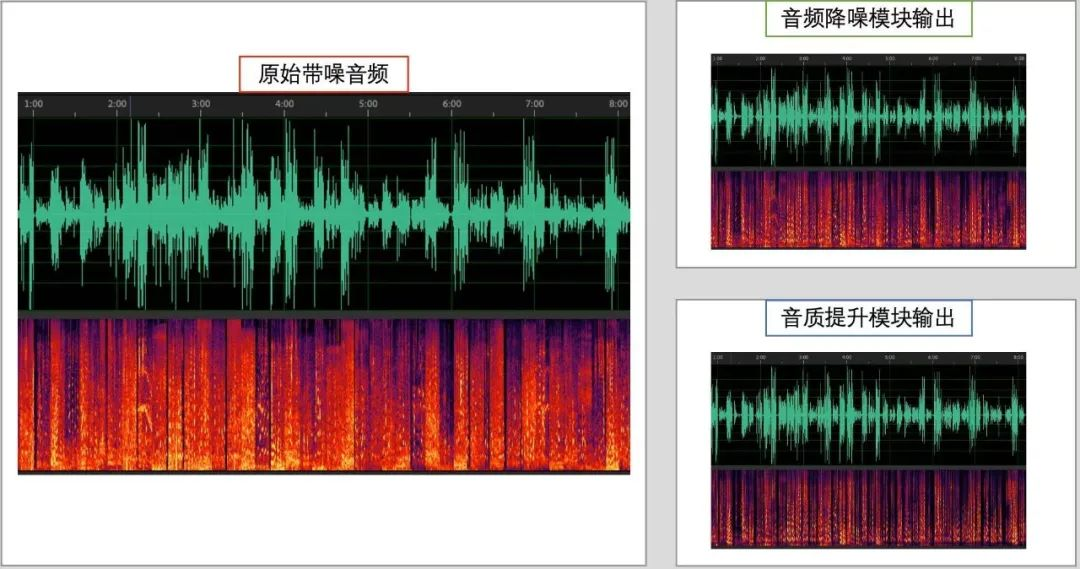Fig.4 音频降噪效果时频图展示

客观指标评估
降噪模型的客观评价指标通过 PESQ(Perceptual evaluation of speech quality)和 STOI(Short-Time Objective Intelligibility)来衡量。我们选取了办公场景下常见的百种噪声：键盘声、关门声、风扇声等噪音，结合干净人声按照 0dB、5dB、10dB 和 15dB 信噪比制作混合音频，用于测试降噪模型的表现。
Tab.2 展示了降噪模型进行蒸馏前后的评估指标对比，AI-Denoise 所在行表示未经过蒸馏技术，正常训练收敛的模型指标，AI-Denoise-teacher 表示放大了参数量的降噪模型，AI-Denoise-student 表示经过蒸馏后与 AI-Denoise 模型相同结构的模型。从表格中看出，AI-Denoise 模型经过蒸馏后在 PESQ 和 STO 指标上均有提升。
Tab.2 AI-Denoise 降噪模型蒸馏前后指标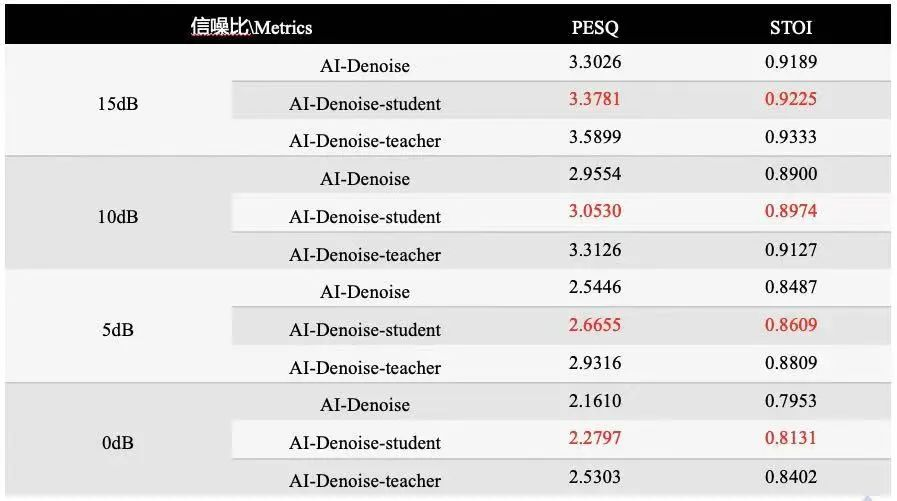目前的降噪模型在多信噪比混合场景下的评估指标，如 Fig.5 所示。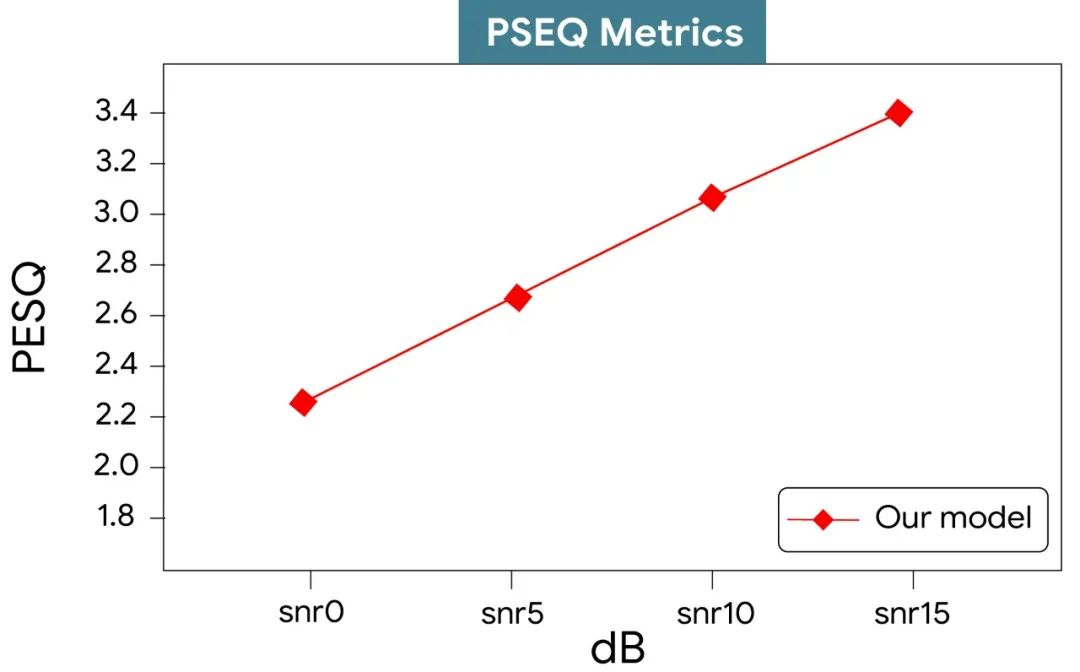Fig.5 降噪模型的评估指标

听觉效果
我们以办公场景噪声为例，在 TensorFlow 公众号文章中展示模型的降噪效果，欢迎大家点击试听。

总结与展望
本文以 TensorFlow 助力：AI 语音降噪打造 QQ 音视频通话新体验为题，介绍了基于 TensorFlow 搭建深度学习模型、TensorFlow Lite 助力模型部署落地的实现过程。
首先介绍了如何基于 TensorFlow 搭建 AI 降噪模型的训练框架，包括数据处理、模型的建立与训练和模型的部署方法；然后，分别介绍降噪模型的音频降噪模块和音质提升模块；继而，通过蒸馏技术优化模型来平衡性能指标与效果评估指标；最后，从视觉、听觉和客观评价指标方面分别展示算法效果。以上功能的实现离不开  Google TensorFlow 生态的支持。
未来将继续在 TensorFlow 这一强大工具的支持下，利用 AI 方法来提升用户在音视频通话时的体验。

参考资料
1. Luo, Yi, and Nima Mesgarani. "Conv-tasnet: Surpassing ideal time–frequency magnitude masking for speech separation." IEEE/ACM transactions on audio, speech, and language processing 27.8 (2019): 1256-1266.
2. Hu Y, Liu Y, Lv S, et al. Dccrn: Deep complex convolution recurrent network for phase-aware speech enhancement[J]. arXiv preprint arXiv:2008.00264, 2020.

想了解更多 TensorFlow Lite 案例，请扫描下方二维码，关注 TensorFlow 官方公众号获取更多信息。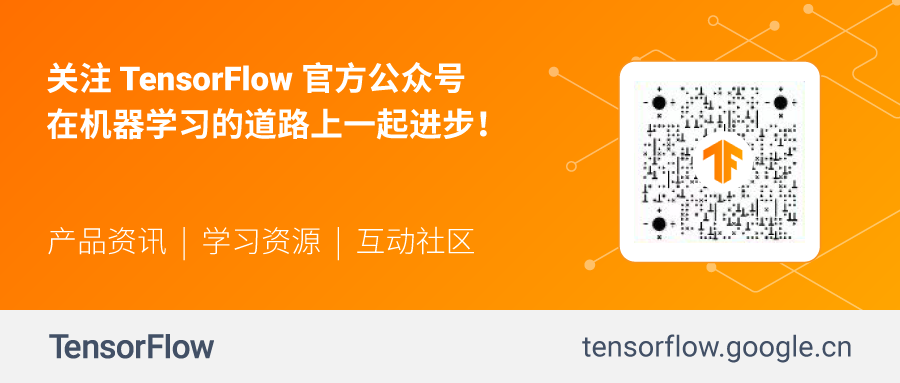展开全文• 上篇(webRTC中语音降噪模块ANS细节详解(二))讲了ANS的处理流程和语音在时域和频域的相互转换。本篇开始讲语音降噪的核心部分，首先讲噪声的初始估计以及基于估计出来的噪声算先验信噪比和后验信噪比。 1，初始...
上篇(webRTC中语音降噪模块ANS细节详解(二) )讲了ANS的处理流程和语音在时域和频域的相互转换。本篇开始讲语音降噪的核心部分，首先讲噪声的初始估计以及基于估计出来的噪声算先验信噪比和后验信噪比。

1，初始噪声估计
webRTC中ANS的初始噪声估计用的是分位数噪声估计法（QBNE，Quantile Based Noise Estimation），对应的论文为《Quantile Based Noise Estimation For Spectral Subtraction And Wiener Filtering》。 分位数噪声估计认为，即使是语音段，输入信号在某些频带分量上也可能没有信号能量，那么将某个频带上所有语音帧的能量做一个统计，设定一个分位数值，低于分位数值的认为是噪声，高于分位数值的认为是语音。算法大致步骤如下：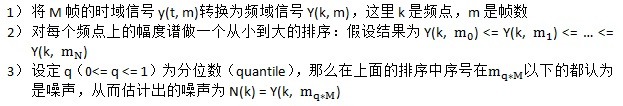webRTC ANS在做初始估计时，分三个阶段，第一个阶段是前50帧，第二个阶段是51~200帧，第三个阶段是200帧以后的。50帧以后的只用分位数噪声估计法来估计噪声，而前50帧是分位数噪声估计法和噪声模型相结合，使噪声估计的更准确。先看每个阶段都有的分位数噪声估计的处理，过程如下：
1）  算出每个频点的幅度谱的自然对数值，即对数谱inst->lmagn，后续用lmagn表示
2）  更新分位数自然对数值(inst->lquantile，后续用lquantile表示)和概率密度值(inst->density，后续用density表示)。 共有三组lquantile和density值，每一帧有129个频点，所以lquantile和density的数组大小为387（129*3）。内存布局示意如图1：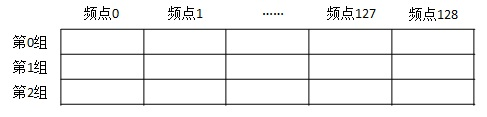图1
三组不同的lquantile和density的更新由inst->counter（后续用counter表示）来控制。counter数组有三个整数值，每个值控制一组。counter数组的初始值基于200（表示前200帧），将200一分为三，即为[66, 133, 200]。每处理完一帧counter值会加1，当值变为200时就会变为0。这样处理第二帧时counter值变为[67, 134, 0]，处理第三帧时counter值变为[68, 135, 1]，以此类推。当初始200帧处理完后，counter也完成了0~200的遍历。

下面看counter怎么控制lquantile和density的，对于第i组第j个频点而言，先定义变量：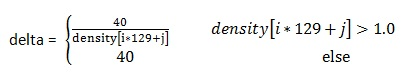更新分位数：当频点对数谱lmagn[j] > lquantile[i*129 + j]时，表示lquantile偏小，需要增大，反之则需要减小。更新数学表达式如下式1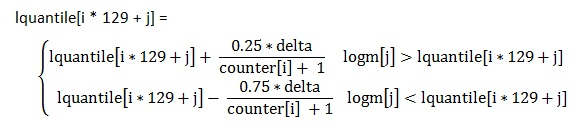（1）
更新概率密度：当|lmagn[j] – lquantile[i*129+j]| < WIDTH(值为0.01)时，意味着当前的噪声估计比较准确了，因此要更新概率密度。更新的数学表达式如下式2：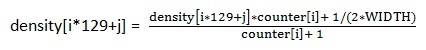3）  当帧数小于200时，对最后一组（即第二组）的lquantile做自然指数运算，将其作为噪声估计值（noise[j]，每个频点一个值），可以看出每帧估出的噪声是不同的。当帧数大于等于200后，只有当counter数组里的值等于200时，才会将对应的组的lquantile做自然指数运算，将其作为噪声估计值。可以看出当帧数大于等于200后每过66帧或者67帧噪声估计值才会更新。

再看前50帧利用分位数噪声估计法与噪声模型相结合来估计初始噪声。先定义如下四个变量：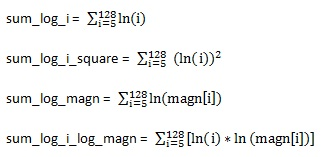需要注意的是上述4个变量定义时均没有用到前5个频点，因为i是从5开始的。再利用上面定义的变量表示白噪声（white noise）和粉红噪声（pink noise）的参数，表示如下：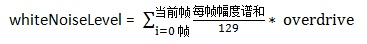其中overdrive是根据设置的降噪程度而得到的一个值（在初始化中设置）。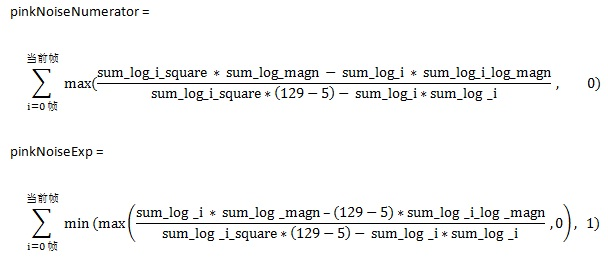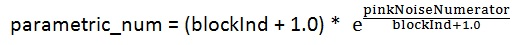其中blockInd表示当前帧的index 。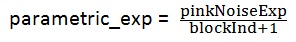这样就可以利用白噪声和粉红噪声的参数来估计模型噪声了，具体如下：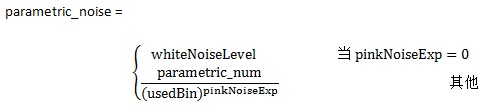其中当频点id小于5时，usedBin = 5, 其他情况下usedBin = 频点id。
最后根据分位数估计噪声noise和模型估计噪声parametric_noise得到最终的估计噪声了。对于每个频点j来说，表达式如下式3: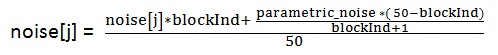(3)
至此，前50帧的结合分位数噪声估计和模型噪声估计的噪声就估计出来了。这样不管是第几帧，初始噪声都能估计出来，下面根据估计出来的初始噪声来算先验信噪比和后验信噪比。

2，算先验信噪比和后验信噪比
在webRTC中语音降噪模块ANS细节详解(一)中说过后验信噪比σ是带噪语音Y与噪声N的功率比值，先验信噪比ρ是干净语音S与噪声N的功率比值，表达式如下式4和5：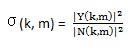(4)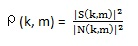(5)
其中m表示第几帧，k表示第几个频点，即每一个频点上都有先验SNR和后验SNR。由于噪声N已通过分位数估计法估计出来，而且带噪语音Y已知，因而后验SNR可以算出来。
因为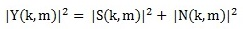从而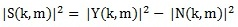所以得到式6：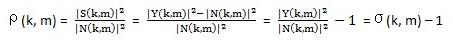(6)
即先验SNR等于后验SNR – 1。
至于算先验SNR，用的是判决引导法（Decision-Directed，简称DD）。根据式5和式6可以得到式7：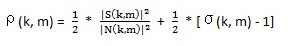(7)
对先验SNR的估算可以将上式递推化得到，具体如式8：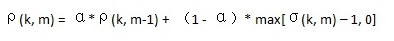(8)
这里α为权重（或叫平滑系数），以代替上式中的1/2。从上式看出估算当前帧的先验SNR是基于上一帧的先验SNR和当前帧的后验SNR。max()用以保证估值是非负的。平滑系数α取值范围为0 < α < 1，典型取值为0.98，webRTC ANS中就是用的这个值。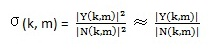(9)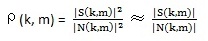(10)
算当前帧的先验SNR时，上一帧的带噪语音Y(k, m-1)是已知的，上一帧的维纳滤波器系数的值H(k, m-1)（即inst-smooth数组里的值）也是已知的，根据维纳滤波原理，从而上一帧的估计出来的干净语音S(k, m-1) = H(k, m-1)Y(k, m-1)也是已知的，所以上一帧的先验SNR计算如式11：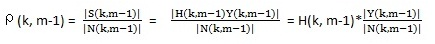(11)
将其带入式8可得当前帧的先验SNR计算表达式如式12：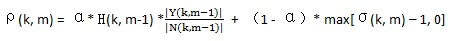(12)
这样当前帧的先验SNR和后验SNR都计算出来了，用于后面的语音噪声概率计算中。下一篇将讲基于带噪语音和特征的语音和噪声的概率计算方法和噪声估计更新以及基于维纳滤波的降噪。
展开全文• 调用speex库进行语音降噪的代码，可以直接运行，直接看到降噪效果
•   谱减法作为语音降噪处理算法中的经典算法，因其运行和处理快，而被广泛应用。 二、谱减法原理   利用带噪信号的频谱减去噪声信号的频谱，讲到这里小伙伴们肯定会有疑问–噪声信号的频谱是怎么得到的呢?请耐心...


谱减法
一、引言二、简单谱减法  2.1 谱减法使用场景  2.2 简单谱减法基本思想  2.3 简单谱减法数学模型  2.4 简单谱减法结果展示
三、经典语音增强方法-过减法  3.1 简单谱减法的缺点  3.2 改进后的谱减法-->过减法  3.2 过减法数学公式  3.3 过减法降噪结果展示
四、参考文献

一、引言
谱减法作为语音降噪处理算法中的经典算法，因其运行和处理快，而被广泛应用。
二、简单谱减法
2.1 谱减法使用场景
1) 噪声为是平稳的(也即在整个时间范围内，噪声的均值和方差基本保持不变)；     2) 噪声为加性噪声。
2.2 简单谱减法基本思想
默认混合信号(含噪信号)的前几帧仅包含环境噪声，并利用混合信号的前几帧的平均幅度谱或者能量谱作为估计到一帧噪声的幅度谱或者能量谱。最后利用混合信号(含噪信号)的幅度谱或者能量谱与估计到的幅度谱与能量谱相减，得到估计到的干净信号的幅度谱或者能量谱。
2.3 简单谱减法数学模型
接收到的含噪语音信号时域数学模型如下：

y

(

n

)

=

x

(

n

)

+

n

(

n

)

y(n)=x(n)+n(n)

其中，

y

(

n

)

y(n)

表示混合信号(含噪信号)，也即待降噪信号；

x

(

n

)

x(n)

表示干净信的语音信号；

n

(

n

)

n(n)

表示噪声信号。其频域表达式如下所示：

Y

(

ω

)

=

X

(

ω

)

+

N

(

ω

)

Y(\omega)=X(\omega)+N(\omega)

出增强后语音信号出现幅度谱负数的情况用0替换，

∣

X

^

(

ω

)

∣

=

{

(

∣

Y

(

ω

)

∣

γ

−

∣

N

^

(

ω

)

∣

∣

∣

1

γ

∣

Y

(

ω

)

∣

γ

−

∣

N

^

(

ω

)

∣

∣

γ

≥

0

0

else

|\hat{X}(\omega)|=\left\{\begin{array}{cc} \left(|Y(\omega)|^{\gamma}-\left.|\hat{N}(\omega)|^{\mid}\right|^{\frac{1}{\gamma}}\right. & |Y(\omega)|^{\gamma}-|\hat{N}(\omega)|^{\mid \gamma} \geq 0 \\ 0 & \text { else } \end{array}\right.

其中，若

γ

=

1

,

∣

Y

(

ω

)

∣

\gamma=1,|Y(\omega)|

为混合信号(含噪信号)的幅度谱;若

γ

=

2

,

∣

Y

(

ω

)

∣

2

\gamma=2,|Y(\omega)|^2

为混合信号(含噪信号)的能量谱;

∣

N

^

(

ω

)

∣

∣

γ

|\hat{N}(\omega)|^{\mid \gamma}

为混合信号(含噪信号)估计到的幅度谱或者能量谱。其系统框图如下所示：简单谱减法代码实现步骤：     1) 初始化参数，确定帧长，帧移，傅里叶变换长度，确定估计噪声谱的帧数     2) 读入混合语音信号，根据帧长和帧移计算帧数。     3) 根据确定用于估计噪声谱的帧数，估计噪声谱。     4) 对每一帧混合信号进行stft变换，然后用含噪信号谱减去噪声谱，得到增强后的信号的幅度谱或者能量谱。     5) 若增强后的信号的幅度谱或者能量谱出现负值，则将其替换为0。     6) 利用混合信号的相位和增强后信号的幅度谱进行重构-反变换到时域。
2.4 简单谱减法结果展示三、经典语音增强方法-过减法
3.1 简单谱减法的缺点
在简单谱减法过程中，如果混合信号的幅度谱与估计出来的噪声谱相减出现负值，说明对噪声出现了过估计问题，仅是简单的将出现的负值设为0，将会导致信号帧频谱的随机位置上出现小的、独立的峰值，称之为音乐噪声。
3.2 改进后的谱减法–>过减法
改进的点如下所示：      1) 在简单谱减法的基础上加上过减因子

α

\alpha

2) 对过减后出现的负值用

β

∣

N

^

(

ω

)

∣

\beta|\hat{N}(\omega)|

替换，

∣

N

^

(

ω

)

∣

|\hat{N}(\omega)|

为估计到的噪声的幅度谱。
3.2 过减法数学公式
改进后的过减法数学公式如下所示，

∣

X

^

(

ω

)

∣

=

(

∣

Y

(

ω

)

∣

γ

−

α

∣

N

^

(

ω

)

∣

γ

)

1

γ

|\hat{X}(\omega)|=\left(|Y(\omega)|^{\gamma}-\alpha|\hat{N}(\omega)|^{\gamma}\right)^{\frac{1}{\gamma}}

出增强后语音信号出现幅度谱负数的情况用

β

∣

N

^

(

ω

)

∣

\beta|\hat{N}(\omega)|

替换，

∣

X

^

(

ω

)

∣

=

{

β

∣

N

^

(

ω

)

∣

∣

X

^

(

ω

)

∣

<

β

∣

N

^

(

ω

)

∣

∣

X

^

(

ω

)

∣

else

|\hat{X}(\omega)|= \begin{cases}\beta|\hat{N}(\omega)| & |\hat{X}(\omega)|<\beta|\hat{N}(\omega)| \\ |\hat{X}(\omega)| & \text { else }\end{cases}

3.3 过减法降噪结果展示四、参考文献
1、语音降噪初探   2、鲁东大学-于泓老师语音增强课程
展开全文机器学习 深度学习
• 一、前言        语音增强，经过近50年的研究发展，涌现出了很多优秀的降噪...自此以后，对于语音降噪的研究在很长一段时间内处于平缓的发展状态，很多的研究都是基于之前提出的...神经网络
• 提出了一种基于仿生小波变换和模糊推理的变步长自适应滤波语音降噪算法。该算法首先用仿生小波变换法对包含噪声的语音信号进行小波分解，以分离出来的噪声信号作为自适应滤波器的输入，选择基于模糊推理变步长自适应...
• SNR越低，降噪效果越差 1.LMS自适应滤波器、陷波器降噪 2.普减法 3.维纳滤波降噪 4.双麦克风降噪 双麦克风，一个放在靠近嘴巴的地方收集人声，一个放在顶端或背部收集环境音，再通过算法将人声从背景音中剥离出来，...
• 语音降噪-谱减算法（改进）过减和最小值保护最优过减因子 过减和最小值保护 谱减法的半波整流（小于0，置0）方法，会引入噪声，为了减少音乐噪声，将满足条件的频点不设置为0，而是设置为相邻几帧的最小值。 ∣Xi^(w...
• 一、获取代码方式 获取代码方式1： 完整代码已上传我的资源：【语音去噪】基于matlab小波硬阈值语音降噪【含Matlab源码 532期】 获取代码方式2： 通过紫极神光博客主页开通CSDN会员，凭支付凭证，私信博主，可获得此...matlab
• 点击查看：Matlab语音降噪源码 文件大小：3M 操作系统：Windows10旗舰版 开发工具：Matlab2016b、2018a 开发语言：.m 点击查看：演示视频 提取码：61ic
• 基于小波变换的语音降噪分析与实现基于小波变换的语音降噪分析与实现基于小波变换的语音降噪分析与实现
• 实时语音通信发展到今天，用户对通话语音质量提出了越来越高的要求。由于终端设备的多样性以及使用场景的差异，声音问题依然存在。传统的音频处理技术从声音信号本身出发，挖掘其时频特性，作出假设，建立物理模型，...
• 近半年来，我利用业余时间在看着《语音增强：理论与实践》和《实时语音处理时间指南》这两本书，对降噪算法有了更深的理解，同时又对ANS的代码进行了调试，基本掌握了算法实现。我想把我对ANS的理解写出来。由于内容...算法
• 基于人工智能的语音降噪方法、装置和计算机设备 技术领域 本申请涉及语音处理技术领域，尤其涉及一种基于人工智能的语音降噪方法、装置和计算机设备。 背景技术 随着语音技术的发展，语音识别在日常生活中...
• 而谱减法作为经典的降噪算法实现简单，运行处理快，被广泛的应用在语音降噪领域。 1. 谱减法原理 谱减法顾名思义，就是用带噪信号的频谱减去噪声信号的频谱（幅度谱和功率谱均可）。谱减法基于一个简单的假设：...
• ## 谱减法语音降噪的Python实现

万次阅读 多人点赞 2017-03-03 16:18:18
谱减法语音降噪的Python实现 本文是参考 [投稿]谱减法语音降噪原理 Matlab版本的代码，采用Python实现的。具体的降噪原理请参阅上文。 下面是谱减法语音降噪的Python实现 文件speech_enhanced.py #!/usr/...python 语音增强
• 浮云降噪是一款智能化的音频增益软件，可实现音频降噪和调高音量等功能。程序采用最先进的人工智能算法，可以极大消除音频中的风声、水声、电流声等多种噪声，与此同时，还可以调高音量，最终导出高品质的音频文件。......